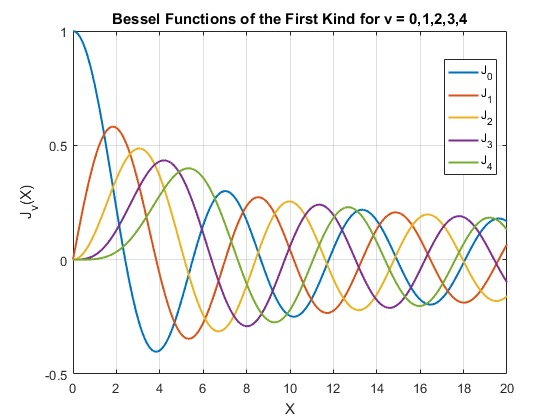## Bessel series for a constant

Fourier series express functions as a sum of sines and cosines of different frequencies. Bessel series are analogous, expressing functions as a sum of Bessel functions of different orders.

Fourier series arise naturally when working in rectangular coordinates. Bessel series arise naturally when working in polar coordinates.

The Fourier series for a constant is trivial. You can think of a constant as a cosine with frequency zero.

The Bessel series for a constant is not as simple, but more interesting. Here we have:$1=J_0(x)+2J_2(x)+2J_4(x)+2J_g(x)\cdots$

Since$J_{-n}=(-1)^n J_n(x)$ we can write the series above as the following infinite series:$1=\sum_{n=-\infty}^{\infty} J_{2n}(x)$

Cool, right?